Ex 5.7

Chapter 5 Class 12 Continuity and Differentiability
Serial order wise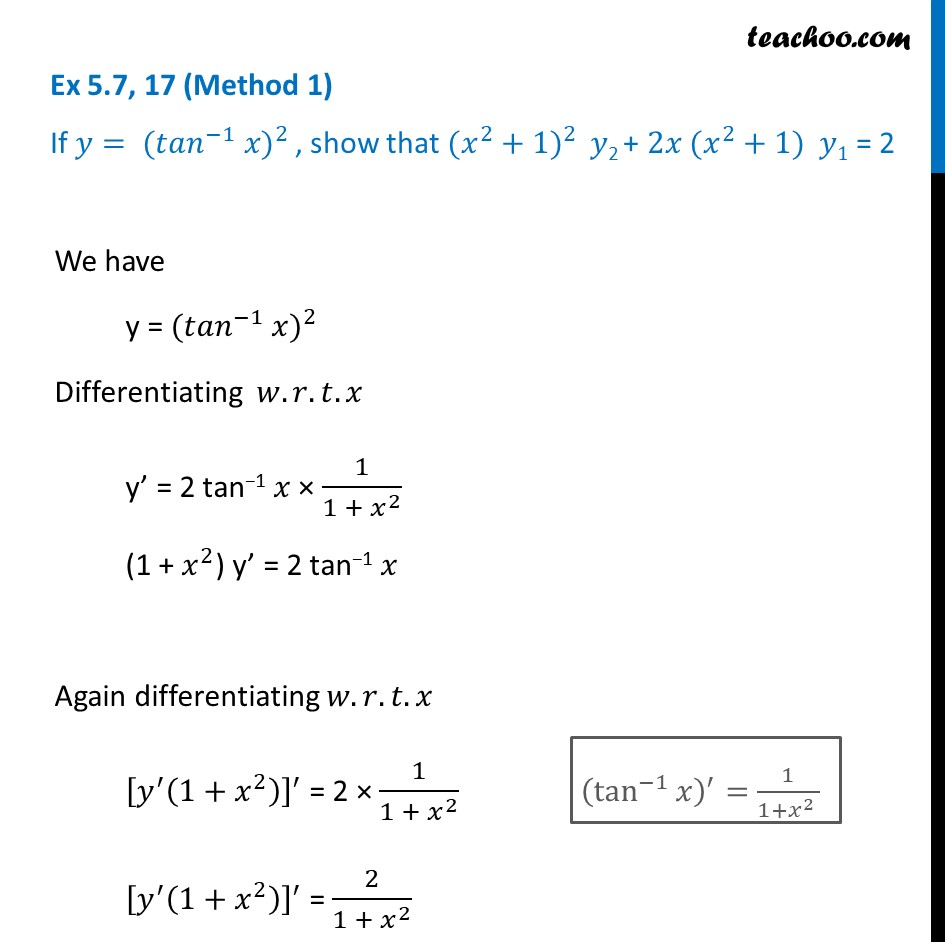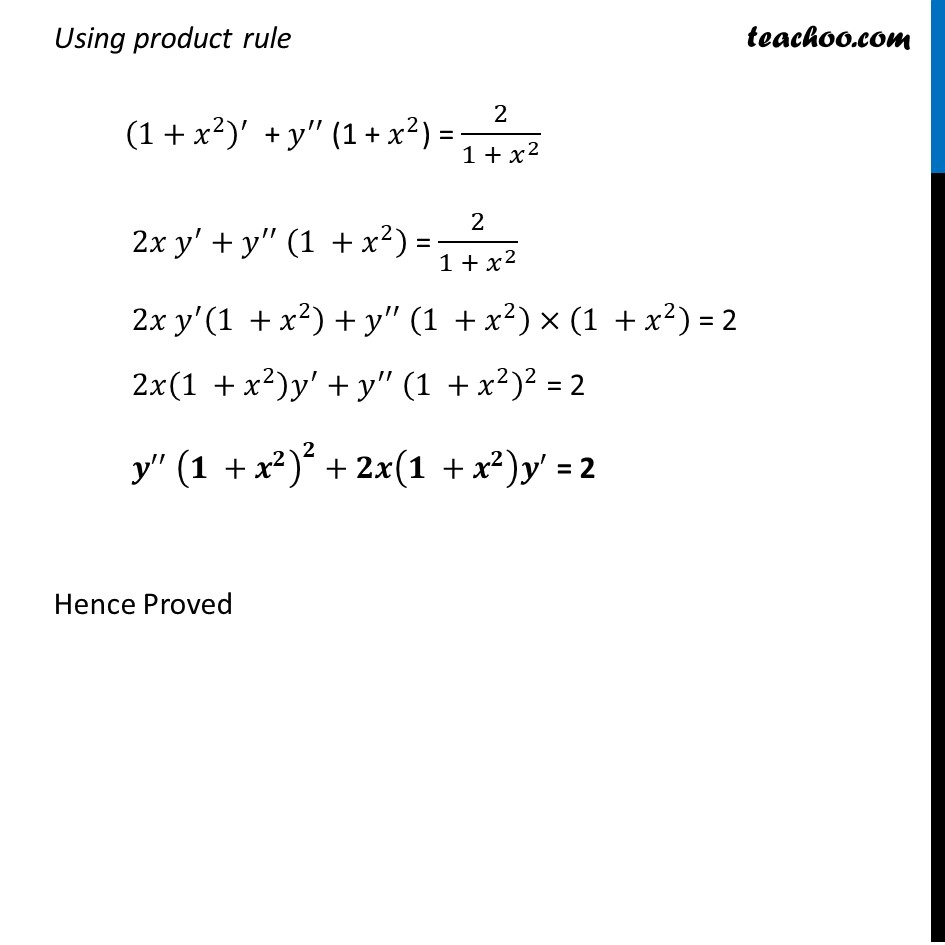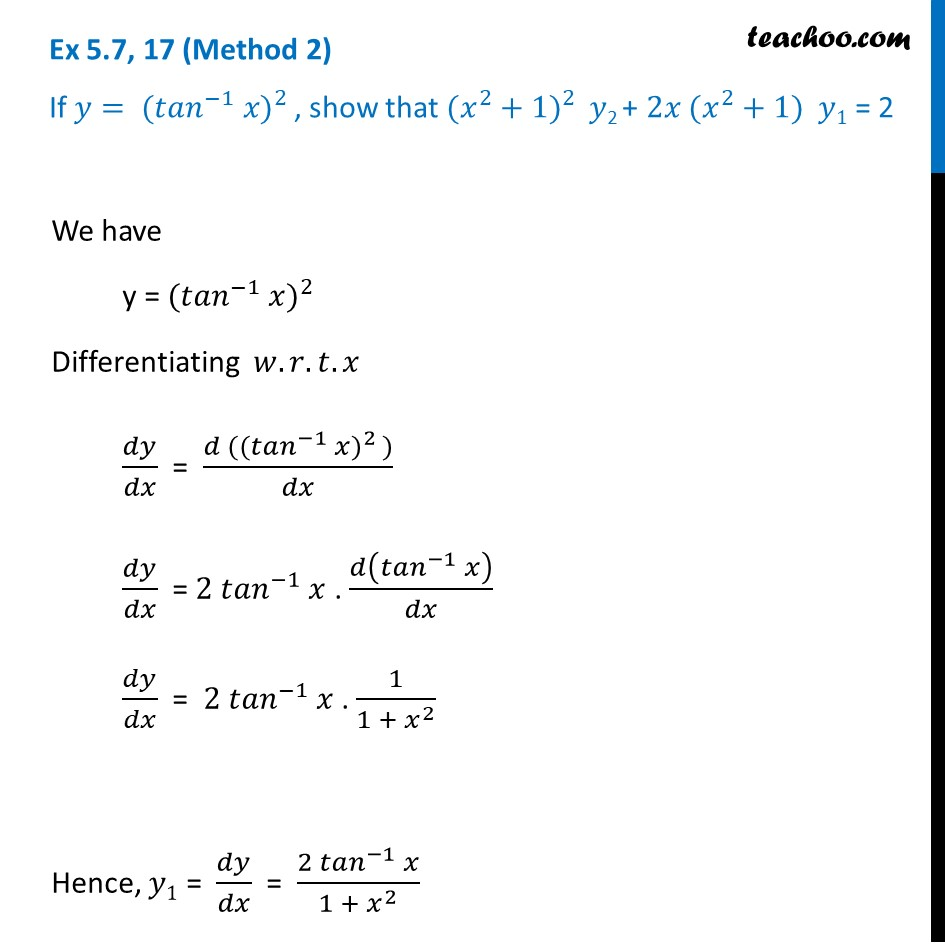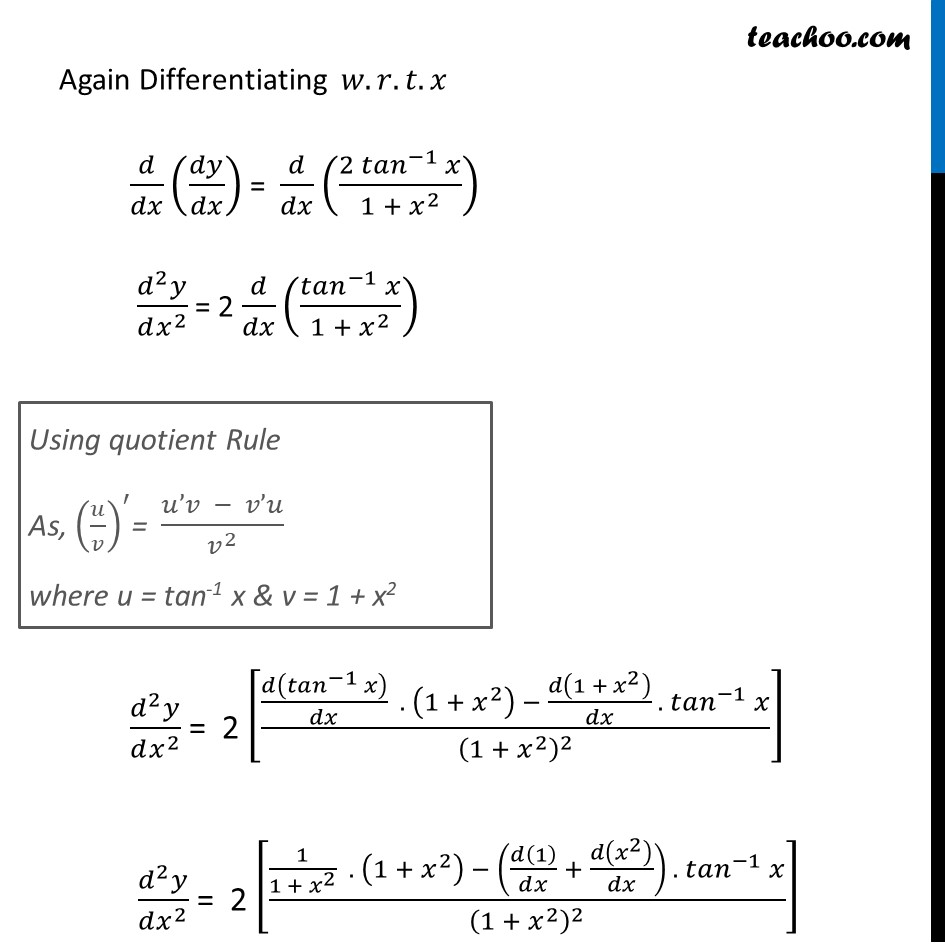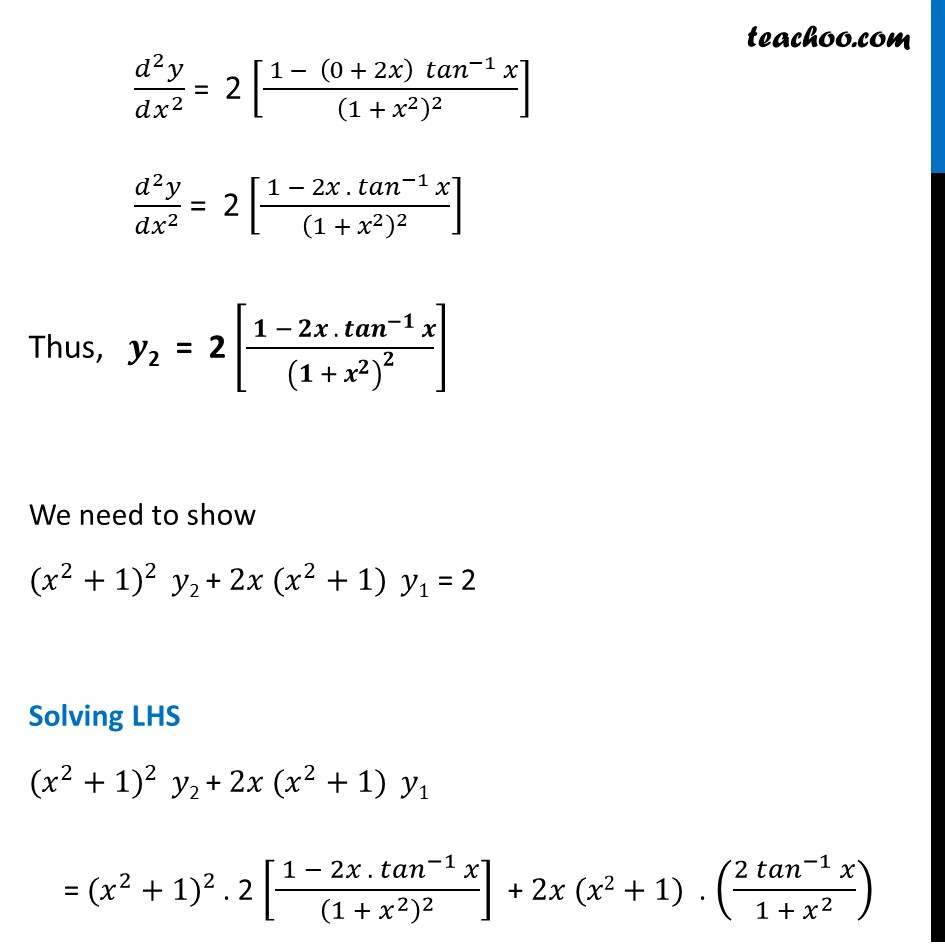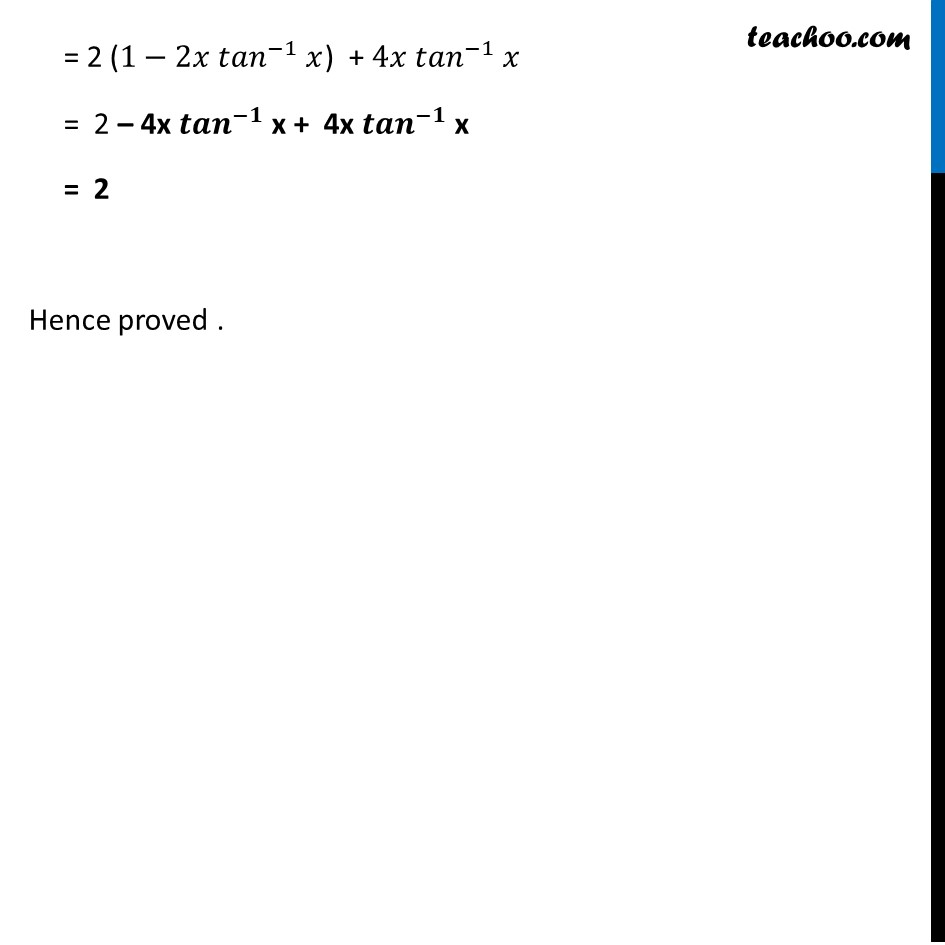Get live Maths 1-on-1 Classs - Class 6 to 12

### Transcript

Ex 5.7, 17 (Method 1) If 𝑦= 〖(〖𝑡𝑎𝑛〗^(−1) 𝑥)〗^(2 ), show that 〖(𝑥^2+1)〗^(2 ) 𝑦2 + 2𝑥 〖(𝑥^2+1)〗^ 𝑦1 = 2 We have y = 〖(〖𝑡𝑎𝑛〗^(−1) 𝑥)〗^(2 ) Differentiating 𝑤.𝑟.𝑡.𝑥 y’ = 2 tan−1 𝑥 × 1/(1 + 𝑥^2 ) (1 + 𝑥^2) y’ = 2 tan−1 𝑥 Again differentiating 𝑤.𝑟.𝑡.𝑥 [𝑦^′ (1+𝑥^2 )]^′ = 2 × 1/(1 +〖 𝑥〗^2 ) [𝑦^′ (1+𝑥^2 )]^′ = 2/(1 + 𝑥^2 ) (tan^(−1)⁡𝑥 )^′=1/(1+𝑥^2 ) Using product rule (1+𝑥^2 )^′ + 𝑦^′′ (1 + 𝑥^2) = 2/(1 + 𝑥^2 ) 2𝑥 𝑦^′+𝑦^′′ (1 +𝑥^2 ) = 2/(1 + 𝑥^2 ) 2𝑥 𝑦^′ (1 +𝑥^2 )+𝑦^′′ (1 +𝑥^2 )×(1 +𝑥^2 ) = 2 2𝑥(1 +𝑥^2 ) 𝑦^′+𝑦^′′ (1 +𝑥^2 )^2 = 2 𝒚^′′ (𝟏 +𝒙^𝟐 )^𝟐+𝟐𝒙(𝟏 +𝒙^𝟐 ) 𝒚^′ = 2 Hence Proved Ex 5.7, 17 (Method 2) If 𝑦= 〖(〖𝑡𝑎𝑛〗^(−1) 𝑥)〗^(2 ), show that 〖(𝑥^2+1)〗^(2 ) 𝑦2 + 2𝑥 〖(𝑥^2+1)〗^ 𝑦1 = 2 We have y = 〖(〖𝑡𝑎𝑛〗^(−1) 𝑥)〗^(2 ) Differentiating 𝑤.𝑟.𝑡.𝑥 𝑑𝑦/𝑑𝑥 = (𝑑 (〖(〖𝑡𝑎𝑛〗^(−1) 𝑥)〗^(2 )))/𝑑𝑥 𝑑𝑦/𝑑𝑥 = 2 〖𝑡𝑎𝑛〗^(−1) 𝑥 . 𝑑(〖𝑡𝑎𝑛〗^(−1) 𝑥)/𝑑𝑥 𝑑𝑦/𝑑𝑥 = 2 〖𝑡𝑎𝑛〗^(−1) 𝑥 . 1/(1 +〖 𝑥〗^2 ) Hence, 𝑦1 = 𝑑𝑦/𝑑𝑥 = (2 〖𝑡𝑎𝑛〗^(−1) 𝑥)/(1 +〖 𝑥〗^2 ) Again Differentiating 𝑤.𝑟.𝑡.𝑥 𝑑/𝑑𝑥 (𝑑𝑦/𝑑𝑥) = 𝑑/𝑑𝑥 ((2 〖𝑡𝑎𝑛〗^(−1) 𝑥)/(1 +〖 𝑥〗^2 )) (𝑑^2 𝑦)/(𝑑𝑥^2 ) = 2 𝑑/𝑑𝑥 ((〖𝑡𝑎𝑛〗^(−1) 𝑥)/(1 +〖 𝑥〗^2 )) (𝑑^2 𝑦)/(𝑑𝑥^2 ) = 2 [(𝑑(〖𝑡𝑎𝑛〗^(−1) 𝑥)/𝑑𝑥 . (1 +〖 𝑥〗^2 ) − 𝑑(1 +〖 𝑥〗^2 )/𝑑𝑥 .〖 𝑡𝑎𝑛〗^(−1) 𝑥)/(1 +〖 𝑥〗^2 )^2 ] (𝑑^2 𝑦)/(𝑑𝑥^2 ) = 2 [(1/(1 +〖 𝑥〗^2 ) . (1 +〖 𝑥〗^2 ) − (𝑑(1)/𝑑𝑥 + 𝑑(𝑥^2 )/𝑑𝑥) . 〖 𝑡𝑎𝑛〗^(−1) 𝑥)/(1 +〖 𝑥〗^2 )^2 ] Using quotient Rule As, (𝑢/𝑣)^′= (𝑢’𝑣 − 𝑣’𝑢)/𝑣^2 where u = tan-1 x & v = 1 + x2 (𝑑^2 𝑦)/(𝑑𝑥^2 ) = 2 [( 1 − (0 + 2𝑥) 〖 𝑡𝑎𝑛〗^(−1) 𝑥)/(1 +〖 𝑥〗^2 )^2 ] (𝑑^2 𝑦)/(𝑑𝑥^2 ) = 2 [( 1 − 2𝑥 .〖 𝑡𝑎𝑛〗^(−1) 𝑥)/(1 +〖 𝑥〗^2 )^2 ] Thus, 𝒚2 = 2 [( 𝟏 − 𝟐𝒙 .〖 𝒕𝒂𝒏〗^(−𝟏) 𝒙)/(𝟏 +〖 𝒙〗^𝟐 )^𝟐 ] We need to show 〖(𝑥^2+1)〗^(2 ) 𝑦2 + 2𝑥 〖(𝑥^2+1)〗^ 𝑦1 = 2 Solving LHS 〖(𝑥^2+1)〗^(2 ) 𝑦2 + 2𝑥 〖(𝑥^2+1)〗^ 𝑦1 = 〖(𝑥^2+1)〗^(2 ). 2 [( 1 − 2𝑥 .〖 𝑡𝑎𝑛〗^(−1) 𝑥)/(1 +〖 𝑥〗^2 )^2 ] + 2𝑥 (𝑥2+1) . ((2 〖𝑡𝑎𝑛〗^(−1) 𝑥)/(1 +〖 𝑥〗^2 )) = 2 (1−2𝑥 〖𝑡𝑎𝑛〗^(−1) 𝑥) + 4𝑥 〖𝑡𝑎𝑛〗^(−1) 𝑥 = 2 – 4x 〖𝒕𝒂𝒏〗^(−𝟏) x + 4x 〖𝒕𝒂𝒏〗^(−𝟏) x = 2 Hence proved .